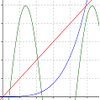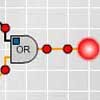# Search by Topic

#### Resources tagged with Mathematical reasoning & proof similar to Spread:

Filter by: Content type:
Age range:
Challenge level:

### There are 176 results

Broad Topics > Using, Applying and Reasoning about Mathematics > Mathematical reasoning & proof### Mind Your Ps and Qs

##### Age 16 to 18 Short Challenge Level:

Sort these mathematical propositions into a series of 8 correct statements.### Gift of Gems

##### Age 14 to 16 Challenge Level:

Four jewellers share their stock. Can you work out the relative values of their gems?### Exponential Intersection

##### Age 16 to 18 Challenge Level:

Can the pdfs and cdfs of an exponential distribution intersect?### Truth Tables and Electronic Circuits

##### Age 11 to 18

Investigate circuits and record your findings in this simple introduction to truth tables and logic.### Logic, Truth Tables and Switching Circuits

##### Age 11 to 18

Learn about the link between logical arguments and electronic circuits. Investigate the logical connectives by making and testing your own circuits and record your findings in truth tables.### Areas and Ratios

##### Age 16 to 18 Challenge Level:

Do you have enough information to work out the area of the shaded quadrilateral?### Big, Bigger, Biggest

##### Age 16 to 18 Challenge Level:

Which is the biggest and which the smallest of $2000^{2002}, 2001^{2001} \text{and } 2002^{2000}$?### Air Nets

##### Age 7 to 18 Challenge Level:

Can you visualise whether these nets fold up into 3D shapes? Watch the videos each time to see if you were correct.### Integral Inequality

##### Age 16 to 18 Challenge Level:

An inequality involving integrals of squares of functions.### Mediant Madness

##### Age 14 to 16 Challenge Level:

Kyle and his teacher disagree about his test score - who is right?### Logic, Truth Tables and Switching Circuits Challenge

##### Age 11 to 18

Learn about the link between logical arguments and electronic circuits. Investigate the logical connectives by making and testing your own circuits and fill in the blanks in truth tables to record. . . .### Napoleon's Hat

##### Age 16 to 18 Challenge Level:

Three equilateral triangles ABC, AYX and XZB are drawn with the point X a moveable point on AB. The points P, Q and R are the centres of the three triangles. What can you say about triangle PQR?### Square Pair Circles

##### Age 16 to 18 Challenge Level:

Investigate the number of points with integer coordinates on circles with centres at the origin for which the square of the radius is a power of 5.### Similarly So

##### Age 14 to 16 Challenge Level:

ABCD is a square. P is the midpoint of AB and is joined to C. A line from D perpendicular to PC meets the line at the point Q. Prove AQ = AD.### Number Rules - OK

##### Age 14 to 16 Challenge Level:

Can you convince me of each of the following: If a square number is multiplied by a square number the product is ALWAYS a square number...### Mouhefanggai

##### Age 14 to 16

Imagine two identical cylindrical pipes meeting at right angles and think about the shape of the space which belongs to both pipes. Early Chinese mathematicians call this shape the mouhefanggai.### Composite Notions

##### Age 14 to 16 Challenge Level:

A composite number is one that is neither prime nor 1. Show that 10201 is composite in any base.### Rhombus in Rectangle

##### Age 14 to 16 Challenge Level:

Take any rectangle ABCD such that AB > BC. The point P is on AB and Q is on CD. Show that there is exactly one position of P and Q such that APCQ is a rhombus.### Pent

##### Age 14 to 18 Challenge Level:

The diagram shows a regular pentagon with sides of unit length. Find all the angles in the diagram. Prove that the quadrilateral shown in red is a rhombus.### A Computer Program to Find Magic Squares

##### Age 16 to 18

This follows up the 'magic Squares for Special Occasions' article which tells you you to create a 4by4 magicsquare with a special date on the top line using no negative numbers and no repeats.### Euler's Formula and Topology

##### Age 16 to 18

Here is a proof of Euler's formula in the plane and on a sphere together with projects to explore cases of the formula for a polygon with holes, for the torus and other solids with holes and the. . . .### Proofs with Pictures

##### Age 14 to 18

Some diagrammatic 'proofs' of algebraic identities and inequalities.### Matter of Scale

##### Age 14 to 16 Challenge Level:

Prove Pythagoras' Theorem using enlargements and scale factors.### The Triangle Game

##### Age 11 to 16 Challenge Level:

Can you discover whether this is a fair game?### Classifying Solids Using Angle Deficiency

##### Age 11 to 16 Challenge Level:

Toni Beardon has chosen this article introducing a rich area for practical exploration and discovery in 3D geometry### Rolling Coins

##### Age 14 to 16 Challenge Level:

A blue coin rolls round two yellow coins which touch. The coins are the same size. How many revolutions does the blue coin make when it rolls all the way round the yellow coins? Investigate for a. . . .### Impossible Sandwiches

##### Age 11 to 18

In this 7-sandwich: 7 1 3 1 6 4 3 5 7 2 4 6 2 5 there are 7 numbers between the 7s, 6 between the 6s etc. The article shows which values of n can make n-sandwiches and which cannot.### Fractional Calculus III

##### Age 16 to 18

Fractional calculus is a generalisation of ordinary calculus where you can differentiate n times when n is not a whole number.### Picturing Pythagorean Triples

##### Age 14 to 18

This article discusses how every Pythagorean triple (a, b, c) can be illustrated by a square and an L shape within another square. You are invited to find some triples for yourself.### Pythagorean Triples I

##### Age 11 to 16

The first of two articles on Pythagorean Triples which asks how many right angled triangles can you find with the lengths of each side exactly a whole number measurement. Try it!### Proof of Pick's Theorem

##### Age 16 to 18 Challenge Level:

Follow the hints and prove Pick's Theorem.### Pythagorean Triples II

##### Age 11 to 16

This is the second article on right-angled triangles whose edge lengths are whole numbers.### Can it Be

##### Age 16 to 18 Challenge Level:

When if ever do you get the right answer if you add two fractions by adding the numerators and adding the denominators?### A Knight's Journey

##### Age 14 to 18

This article looks at knight's moves on a chess board and introduces you to the idea of vectors and vector addition.### Telescoping Functions

##### Age 16 to 18

Take a complicated fraction with the product of five quartics top and bottom and reduce this to a whole number. This is a numerical example involving some clever algebra.### Where Do We Get Our Feet Wet?

##### Age 16 to 18

Professor Korner has generously supported school mathematics for more than 30 years and has been a good friend to NRICH since it started.### Yih or Luk Tsut K'i or Three Men's Morris

##### Age 11 to 18 Challenge Level:

Some puzzles requiring no knowledge of knot theory, just a careful inspection of the patterns. A glimpse of the classification of knots and a little about prime knots, crossing numbers and. . . .### Magic Squares II

##### Age 14 to 18

An article which gives an account of some properties of magic squares.### The Pillar of Chios

##### Age 14 to 16 Challenge Level:

Semicircles are drawn on the sides of a rectangle. Prove that the sum of the areas of the four crescents is equal to the area of the rectangle.### Modulus Arithmetic and a Solution to Differences

##### Age 16 to 18

Peter Zimmerman, a Year 13 student at Mill Hill County High School in Barnet, London wrote this account of modulus arithmetic.### Sums of Squares and Sums of Cubes

##### Age 16 to 18

An account of methods for finding whether or not a number can be written as the sum of two or more squares or as the sum of two or more cubes.### Transitivity

##### Age 16 to 18

Suppose A always beats B and B always beats C, then would you expect A to beat C? Not always! What seems obvious is not always true. Results always need to be proved in mathematics.### More Sums of Squares

##### Age 16 to 18

Tom writes about expressing numbers as the sums of three squares.### Modulus Arithmetic and a Solution to Dirisibly Yours

##### Age 16 to 18

Peter Zimmerman from Mill Hill County High School in Barnet, London gives a neat proof that: 5^(2n+1) + 11^(2n+1) + 17^(2n+1) is divisible by 33 for every non negative integer n.### The Frieze Tree

##### Age 11 to 16

Patterns that repeat in a line are strangely interesting. How many types are there and how do you tell one type from another?### Continued Fractions II

##### Age 16 to 18

In this article we show that every whole number can be written as a continued fraction of the form k/(1+k/(1+k/...)).### Euclid's Algorithm II

##### Age 16 to 18

We continue the discussion given in Euclid's Algorithm I, and here we shall discover when an equation of the form ax+by=c has no solutions, and when it has infinitely many solutions.### Round and Round

##### Age 14 to 16 Challenge Level:

Prove that the shaded area of the semicircle is equal to the area of the inner circle.### Generally Geometric

##### Age 16 to 18 Challenge Level:

Generalise the sum of a GP by using derivatives to make the coefficients into powers of the natural numbers.### Pareq Exists

##### Age 14 to 16 Challenge Level:

Prove that, given any three parallel lines, an equilateral triangle always exists with one vertex on each of the three lines.